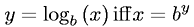Equations > Algebra > Logarithms > Definition of a Logarithm

### Definition of a LogarithmLatex Code:

MathML Code:

 $y={\mathrm{log}}_{b}\left(x\right)\mathrm{iff}x={b}^{y}$

MathType 5.0: